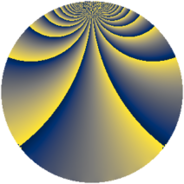# Properties

 Label 1600.2.uLevel $1600$ Weight $2$ Character orbit 1600.u Rep. character $\chi_{1600}(321,\cdot)$ Character field $\Q(\zeta_{5})$ Dimension $232$ Sturm bound $480$

# Related objects

## Defining parameters

 Level: $$N$$ $$=$$ $$1600 = 2^{6} \cdot 5^{2}$$ Weight: $$k$$ $$=$$ $$2$$ Character orbit: $$[\chi]$$ $$=$$ 1600.u (of order $$5$$ and degree $$4$$) Character conductor: $$\operatorname{cond}(\chi)$$ $$=$$ $$25$$ Character field: $$\Q(\zeta_{5})$$ Sturm bound: $$480$$

## Dimensions

The following table gives the dimensions of various subspaces of $$M_{2}(1600, [\chi])$$.

Total New Old
Modular forms 1008 248 760
Cusp forms 912 232 680
Eisenstein series 96 16 80

## Trace form

 $$232q + 8q^{5} - 60q^{9} + O(q^{10})$$ $$232q + 8q^{5} - 60q^{9} + 6q^{13} - 14q^{17} + 24q^{21} - 12q^{25} + 6q^{29} + 6q^{33} + 6q^{37} - 14q^{41} + 16q^{45} + 168q^{49} + 54q^{53} - 4q^{57} + 6q^{61} - 18q^{65} + 42q^{69} + 2q^{73} + 96q^{77} - 36q^{81} + 22q^{85} - 18q^{89} + 52q^{93} + 34q^{97} + O(q^{100})$$

## Decomposition of $$S_{2}^{\mathrm{new}}(1600, [\chi])$$ into newform subspaces

The newforms in this space have not yet been added to the LMFDB.

## Decomposition of $$S_{2}^{\mathrm{old}}(1600, [\chi])$$ into lower level spaces

$$S_{2}^{\mathrm{old}}(1600, [\chi]) \cong$$ $$S_{2}^{\mathrm{new}}(25, [\chi])$$$$^{\oplus 7}$$$$\oplus$$$$S_{2}^{\mathrm{new}}(50, [\chi])$$$$^{\oplus 6}$$$$\oplus$$$$S_{2}^{\mathrm{new}}(100, [\chi])$$$$^{\oplus 5}$$$$\oplus$$$$S_{2}^{\mathrm{new}}(200, [\chi])$$$$^{\oplus 4}$$$$\oplus$$$$S_{2}^{\mathrm{new}}(400, [\chi])$$$$^{\oplus 3}$$$$\oplus$$$$S_{2}^{\mathrm{new}}(800, [\chi])$$$$^{\oplus 2}$$